Courses

# Test: Rate Law, Molecularity & Order of Reaction

## 20 Questions MCQ Test Chemistry for JEE | Test: Rate Law, Molecularity & Order of Reaction

Description
This mock test of Test: Rate Law, Molecularity & Order of Reaction for JEE helps you for every JEE entrance exam. This contains 20 Multiple Choice Questions for JEE Test: Rate Law, Molecularity & Order of Reaction (mcq) to study with solutions a complete question bank. The solved questions answers in this Test: Rate Law, Molecularity & Order of Reaction quiz give you a good mix of easy questions and tough questions. JEE students definitely take this Test: Rate Law, Molecularity & Order of Reaction exercise for a better result in the exam. You can find other Test: Rate Law, Molecularity & Order of Reaction extra questions, long questions & short questions for JEE on EduRev as well by searching above.
QUESTION: 1

### Direction (Q. Nos. 1-13) This section contains multiple choice questions. Each question has four choices (a), (b), (c) and (d), out of which ONLY ONE is correct Q. In the following reaction, which has maximum rate w.r.t. rate of disappearance of NH3? 4NH3 + 502 →  4NO + 6H2O

Solution:

By stoichiometry of the reaction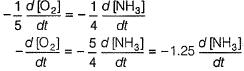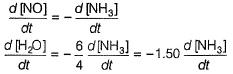QUESTION: 2

### The rate of formation of NO(g) in the reaction, 2NOBr(g) → 2NO(g) + Br2(g) was reported as 1.6 x 10-4 Ms-1. Thus, rate of the reaction is

Solution: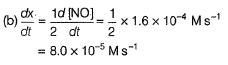QUESTION: 3

### Rate of formation of SO3 in the reaction below is 100 kg min-1. 2SO2 + O2 → 2SO3 Hence, rate of disappearance of SO2 will be:

Solution: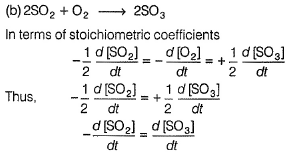Rate of disappearance of SO2 = Rate of formation of SO3 But quantity is to be expressed in terms of moles.
Rate of formation of SO3 = 100 kg SO3 min-1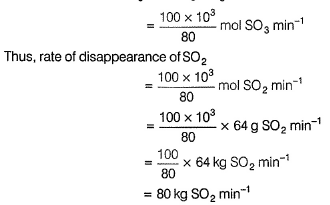QUESTION: 4

The following reaction can take place in both directions: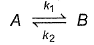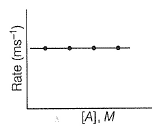For the forward reaction, the rate varies with the concentration of A as and for the backward reaction,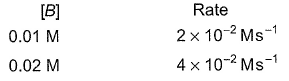Hence, net reaction rate is:

Solution: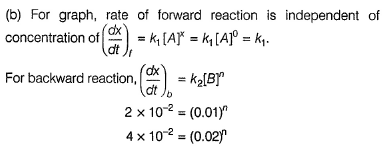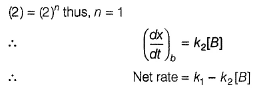QUESTION: 5

For a reaction, xA → yB, rate of disappearance of ‘A’ is related to the rate of appearance of 'B' by the equation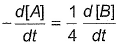Thus, x and y respectively are

Solution: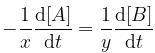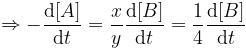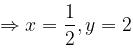QUESTION: 6

For the reaction,

H2(g) + A2(g)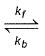2H(g) + A2(g)

kf = 2.2 x 104 L mol-1s-1 and Kc = 1.00 x 10-4 at 3000 K. Thus, kb is

Solution: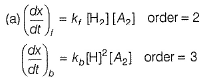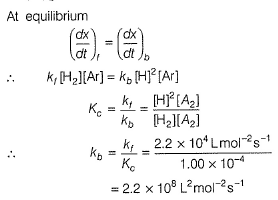QUESTION: 7

For a reaction,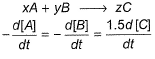hen x, y and z are

Solution:

For the reaction: xA + yB → zC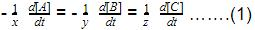Given that,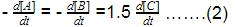Multiplying equation 2 by 1/3, we get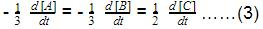On comparing equation 1 and 3,
x = 3, y = 3, z = 2.

QUESTION: 8

For the reaction,

2N2O5(g) → 4NO2 (g) + O2(G)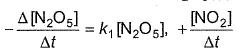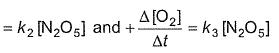Thus,

Solution: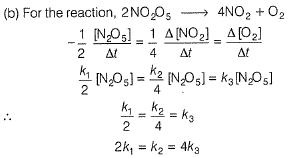QUESTION: 9

The decomposition of acetaldehyde is given by the following reaction:

CH3CHO(g) → CH4(g) + CO(g)

Rate of the reaction with respect to the pressure of the reactant is

Solution: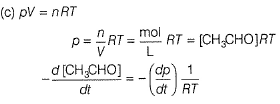QUESTION: 10

For the reaction,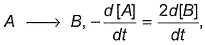then rate law is

Solution: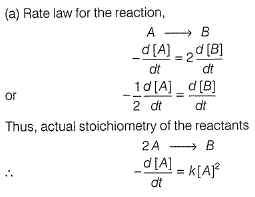QUESTION: 11

For gaseous reaction, the rate can be expressed as

Solution: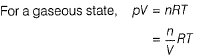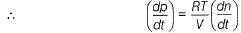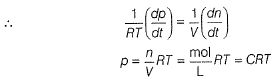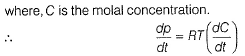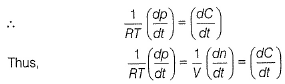QUESTION: 12

The initial rates of reaction for the equation, 2A + B → Products.

Products were determined under various initial concentrations of reactants.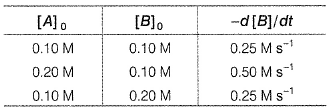Thus, rate law is equal to

Solution:

Let order w.r.t. A = a, order w.r.t. B = b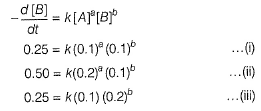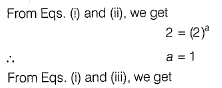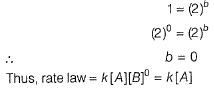QUESTION: 13

For the reaction, A + 2B → Product, the reaction rate was halved on doubling the concentration of A. Thus, order w.r.t. A is

Solution:

If concentration of the nth order reaction is made m time, rate becomes mn times.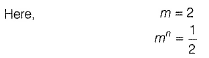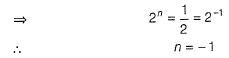QUESTION: 14

Direction (Q. Nos. 14 and 15) This section contains a passage describing theory, experiments, data, etc. Two questions related to the paragraph have been given. Each question has only one correct answer out of the given 4 options (a), (b), (c) and (d)

Passage

The decomposition of NO2 at 400 K proceeds at a of rate of 5.4 x 10 -5 mol L-1 s-1 when [NO2] = 0.01 mol-1

2 NO2(g) → 2NO(g ) + O2(g).

Q. What is the rate law when observed rate is 1.35 x 10-5 mol L-1 s-1 at [NO2] = 0.005 mol L-1?

Solution:

Given unit of rate = mol L-1 s-1
General formula for unit of rate = mol n-1 Ln-1 s -1
On equating,
1-n = 1 ⇒ n = 0
n-1 = -1 ⇒ n = 0

Hence, it is a zero order reaction.

QUESTION: 15

The decomposition of NO2 at 400 K proceeds at a of rate of 5.4 x 10 -5 mol L-1 s-1 when [NO2] = 0.01 mol-1

2 NO2(g) → 2NO(g ) + O2(g).

Q. Rate constant of the reaction will be

Solution: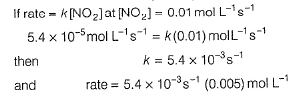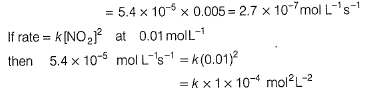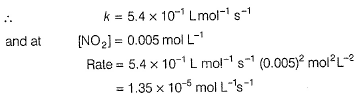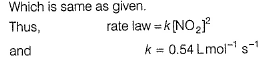QUESTION: 16

Direction (Q. Nos. 16-18) This section contains 3 questions. When worked out will result in one integer from 0 to 9 (both inclusive)

In the following reaction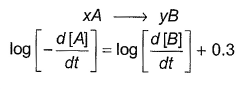Q. Where negative sign indicates rate of disappearance of the reactant. What is the value of x/y ?

Solution: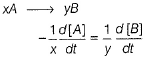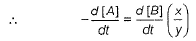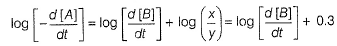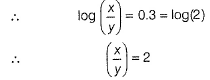QUESTION: 17

The reaction rate is defined as the rate at which the concentration of the reactants __________ with time or the concentration of products ___________ with time.

Solution:

The reaction rate is defined as the rate at which the concentration of the reactants decreases with time or the concentration of products increases with time.

QUESTION: 18

In a certain polluted atmosphere containing O3 at a steady concentration of 2.0 x 10-6 M, the hourly production of O3 by all sources was estimated as 7.2 x 10-15 M. If the only mechanism for destruction of O3 in the second-order reaction is

2O3 → 3O2

then rate constant for destruction reaction, defined by the rate law for - Δ[O3]/Δf is x * 10-7 M-1 s-1 . What is the value of x?

Solution: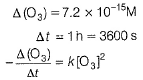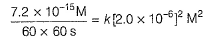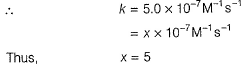QUESTION: 19

Only One Option Correct Type

This section contains 2 multiple choice questions. Each question has four choices (a), (b), (c) and (d), out of which ONLY ONE is correct

For a reversible reaction, net rate is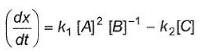hence given reaction is

Solution:

2A - B →(reversible) C

For a forward reaction, (dx/dt)f = k1[A]2 [B]-1
For a backward reaction, (dx/dt)b = k2[C]

Net rate = (dx/dt)f - (dx/dt)= k1[A]2 [B]-1 - k2[C]

QUESTION: 20

Reaction kinetics deals with the study of

Solution:

Reaction kinetics deals with the study of rate of reaction, their mechanism and the factors which affects the rate of reaction. It specifies all the general characteristics of a chemical reaction.